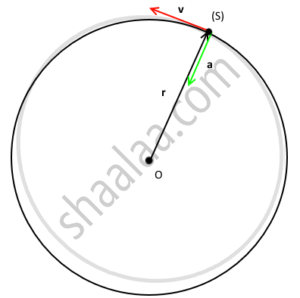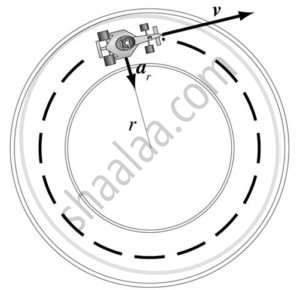# Uniform Circular Motion (UCM)

• Circular motion
• Terms involved in a circular motion
1) Angular displacement
2) Angular Velocity
3) Angular Acceleration
• Uniform Circular Motion (UCM)
• Time Period of UCM
• Frequency of UCM
1) For non-uniform circular motion
• Centripetal force
• Centrifugal force

## Notes

### Circular Motion

Circular motion is the movement of an object in a circular path.#### Uniform Circular Motion

This motion refers to the circular motion if the magnitude of the velocity of the particle in circular motion remains constant. The non-uniform circular motion refers to the circular motion when the magnitude of the velocity of the object is not constant. Another special kind of circular motion is when an object rotates around itself also known by spinning motion.Variables in Circular Motion:-
Angular Displacement
"The angle which is subtended by the position vector at the centre of the circular path refers to the angular displacement."

Angular Displacement (Δθ) = `(ΔS)/(r)`
where Δ’s refers to the linear displacement while r is the radius. Radian is the unit of Angular Displacement.

Angular Acceleration
"It refers to the rate of time of change of angular velocity (dῶ)."

Angular acceleration (α) = `(dῶ)/dt` = `(d^2θ) / (dt^2)`
Its unit is `(rad)/(s^2)` and dimensional formula `[T^(-2)]`. The relation between linear acceleration (a) and angular acceleration (α)
A = rα, where r is the radius.

Angular Velocity
"It refers to the time rate change of angular displacement (dῶ)."

Angular Velocity (ῶ) = `(Δθ)/(Δt)`
Angular Velocity is a vector quantity. Its unit is rad/s. The relation between the linear velocity (v) and angular velocity (ῶ) is
v = rῶ

Centripetal Acceleration
"It refers to an acceleration that acts on the body in circular motion whose direction is always towards the centre of the path."

Centripetal Acceleration (α) = `v^2/r = rῶ^2`.
The magnitude of this acceleration by comparing ratios of velocity and position around the circle. Since the particle is travelling in a circular path, the ratio of the change in velocity to velocity will be the same as the ratio of the change in position to position. It is also known as radial acceleration as it acts along the radius of the circle. Centripetal Acceleration is a vector quantity and the unit is in `m/s^2`.

If you would like to contribute notes or other learning material, please submit them using the button below.

#### Video Tutorials

We have provided more than 1 series of video tutorials for some topics to help you get a better understanding of the topic.

Series 1

Series 2

### Shaalaa.com

Uniform Circular Motion [00:43:31]
S
0%